Courses

# Linear Transform MCQ - 1

## 30 Questions MCQ Test Topic-wise Tests & Solved Examples for IIT JAM Mathematics | Linear Transform MCQ - 1

Description
This mock test of Linear Transform MCQ - 1 for Mathematics helps you for every Mathematics entrance exam. This contains 30 Multiple Choice Questions for Mathematics Linear Transform MCQ - 1 (mcq) to study with solutions a complete question bank. The solved questions answers in this Linear Transform MCQ - 1 quiz give you a good mix of easy questions and tough questions. Mathematics students definitely take this Linear Transform MCQ - 1 exercise for a better result in the exam. You can find other Linear Transform MCQ - 1 extra questions, long questions & short questions for Mathematics on EduRev as well by searching above.
QUESTION: 1

Solution:
QUESTION: 2

Solution:
QUESTION: 3

###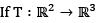is a linear transformation T(1,0) = (2,3,l) and T(1,1) = (3,0,2) then which one of the following statement is correct?

Solution:
QUESTION: 4

Let T:R2 -> R2 be the transformation T(x1,x2) = (x1,0). The null space (or kernel) N(T) of T is

Solution:
QUESTION: 5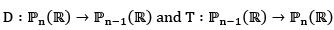defined by differentiation and integration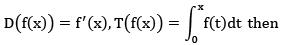Solution:
QUESTION: 6

Which of the following is not linear?

Solution:
QUESTION: 7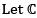be the vector space of all complex numbers over complex field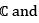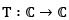be defined by T(z) =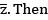Solution:
QUESTION: 8

Consider the vector space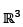and the maps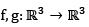defined by f(x,y,z) = (x, |y|,z) and g(x,y,z) = (x + 1,y - 1, z).Then

Solution:

QUESTION: 9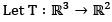be the linear transformation given by T(x,y, z) = (x,y) with respect to standard basis of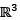and the basis {(0,1). (1,1)} of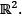What is the matrix representation of T?

Solution:
QUESTION: 10

Define T on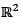into it self by T(x1, x2) = (x1 + x2, x1 - x2).Then matrix of T-1 relative to the standard basis forSolution:

QUESTION: 11

Which of the following is a linear transformation from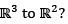(I)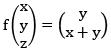(II)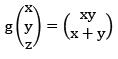(III)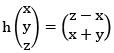Solution:

QUESTION: 12

Consider the basis S = {v1, v2, v3} for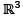where v1 = (1,1,1) and v2 = (1,1,0), v3 = (1,0,0) and let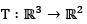be a linear transformation such that T(v1) = (1,0), T (v2) = (2, -1), T (v3) = (4, 3). Then T (2, - 3, 5) is

Solution:
QUESTION: 13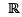is set of real numbers in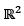let f1 and f2 be the two transformation defined by f1(x,y) = (0,y), f2(x,y) = (y,x). Then the product of the mapping (f2 ° f1)(x,y) gives the projection of the x - y plane on the

Solution:

QUESTION: 14

Consider the following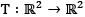such that T(2,2) = (8, - 6), T (5, 5) = (3, - 2) Then

Solution:
QUESTION: 15

Let T be linear transformation on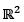into itself such that T(1,0) = (1,2) and T (1, 1) = (0, 2) .Then T(a, b) is equal to

Solution:
QUESTION: 16

Which of the following mapping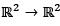is not a linear mapping?

Solution:
QUESTION: 17

If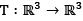is given by T (x, y, z) = (x - y, y + 3z, x + 2y). Then T-1 is

Solution:
QUESTION: 18

Let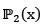be the vector space of all polynomials over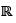of degree less than or equal to 2. Let D be the differential operator on. Then m atrix of D relative to the basis (x2, 1,x} is equal to

Solution:
QUESTION: 19

The matrix of the linear tranformation T on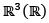defined as T(x,y,z) = (2y + z, x - 4y, 3x) with respect to the basis β = {(1,1,1), (1,1,0), (1,0,0)} is

Solution:
QUESTION: 20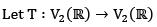where T be the reflection of the points through the line y = -x then the matrix of T with respect to standard basis is

Solution:
QUESTION: 21

Consider the two linear mapsT1 and T2 on V3 defined as T1(x1, x2, x3) = (0, x2, x3) and T2(x1, x2, x3) = (x1, 0,0)

Solution:
QUESTION: 22

Let T be a linear transformation on the vector space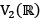defined by T(a, b) = (a, 0) the matrix of T relative to the ordered basis {(1,0), (0,1)} of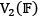is

Solution:
QUESTION: 23

Let W be the vector space of all real polynomials of degree atmost 3. Define T : W → W by T(p(x)) = p'(x) where p'(x) is the derivative of P.The matrix of T in the basis {1, x, x2, x3} considered as column vector is given by

Solution:
QUESTION: 24

Let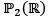be the polynomial space with basis {1, x, x2} then matrix representation of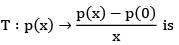Solution:
QUESTION: 25

Let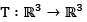be a linear transformation defined by T(x, y, z) = (x + y - z, x + z, y - z) then the matrix of the linear transformation T with respect to ordered basis β = {(0,1,0), (0,0,1), (1,0,0)} of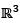is

Solution:

QUESTION: 26

Let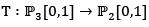be defined by T(p(x)) = p"(x) + p'(x). Then the matrix representation of T with respect to basis {1, x, x2, x3} and {1, x, x2} of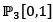and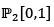respectively is

Solution:
QUESTION: 27

For the standard basis {(1,0,0), (0,1,0), (0,0,1)} of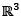is a linear transformation T from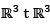has the matrix representation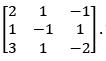Then the image of (2,1,2) under T is

Solution:
QUESTION: 28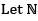be the vector space of all real polynomials of degree atmost 3. Define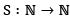then the matrix of S in the basis {1,x,x2,x3}. Considered as column vector is given by

Solution:

QUESTION: 29

Let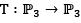be the map given by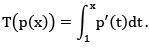If the matrix of T relative to the standard basis β = γ = {1, x, x2, x3} is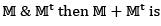Solution:
QUESTION: 30

A linear transformation T rotates each vector in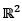clockwise through 90°. The matrix T relative to standard ordered basis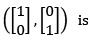Solution: